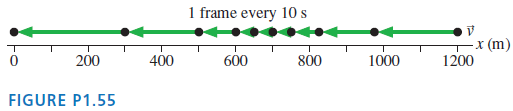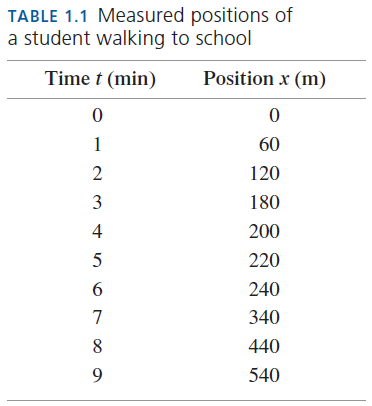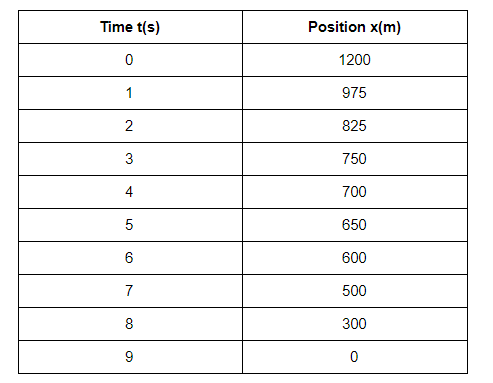# Figure P2.1 shows a motion diagram of a car traveling down

Chapter 1, Problem 55P

(choose chapter or problem)

Get Unlimited Answers! Check out our subscriptions
QUESTION:

Figure P1.55 shows a motion diagram of a car traveling down a street. The camera took one frame every $$10 s$$. A distance scale is provided.a. Measure the $$x$$-value of the car at each dot. Place your data in a table, similar to Table 1.1, showing each position and the instant of time at which it occurred.

b. Make a position-versus-time graph for the car. Because you have data only at certain instants of time, your graph should consist of dots that are not connected together.Equation Transcription:Text Transcription:

10 s

x-value

QUESTION:

Figure P1.55 shows a motion diagram of a car traveling down a street. The camera took one frame every $$10 s$$. A distance scale is provided.a. Measure the $$x$$-value of the car at each dot. Place your data in a table, similar to Table 1.1, showing each position and the instant of time at which it occurred.

b. Make a position-versus-time graph for the car. Because you have data only at certain instants of time, your graph should consist of dots that are not connected together.Equation Transcription:Text Transcription:

10 s

x-value

Step 1 of 2

Part (a)

Our aim is to prepare the table containing the position of the car at each second as given in the diagram.

The measured positions of the car